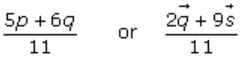# RD Sharma Solutions Class 12 Algebra Of Vectors Exercise 23.3

RD Sharma Solutions for Class 12 Maths Chapter 23 Algebra of Vectors Exercise 23.3 are provided here. Top tutors of BYJU’S have designed these solutions to help students ace their Mathematics exams. These solutions are presented in a simple, precise and step-wise manner to aid all the students in scoring a high score in the subject. The RD Sharma Solutions PDF can be downloaded from the link attached below.

## Download PDF of Rd Sharma Solution for Class 12 Maths Chapter 23 Exercise 3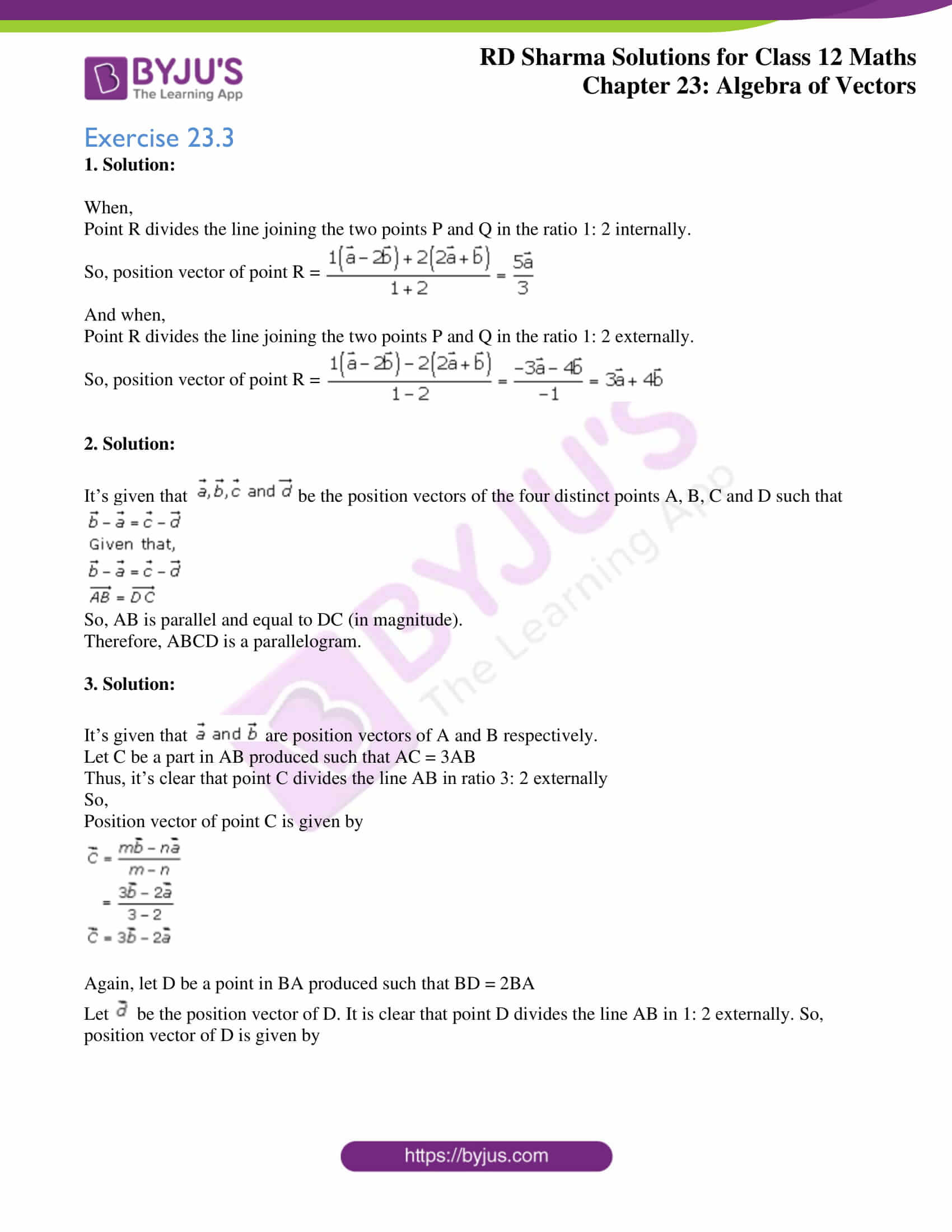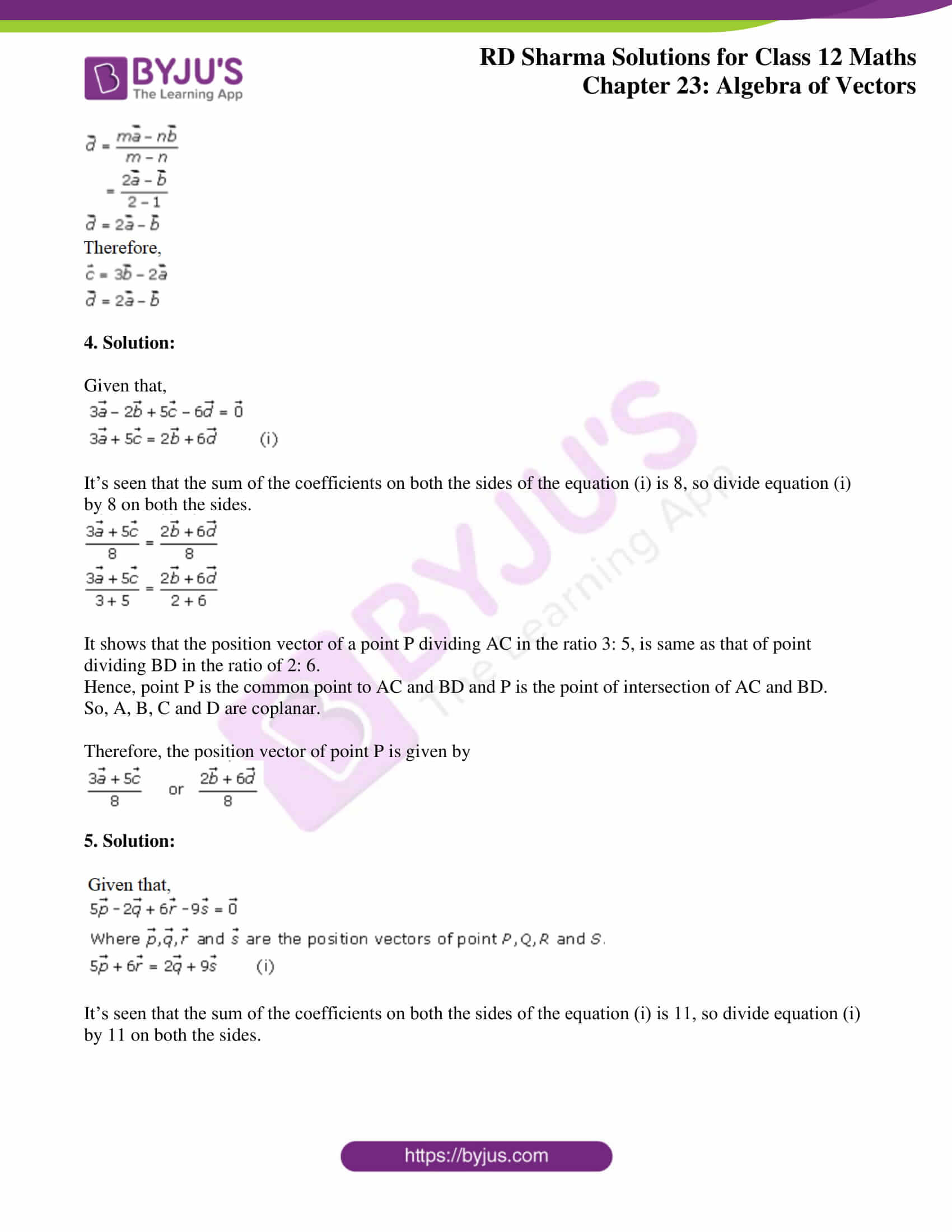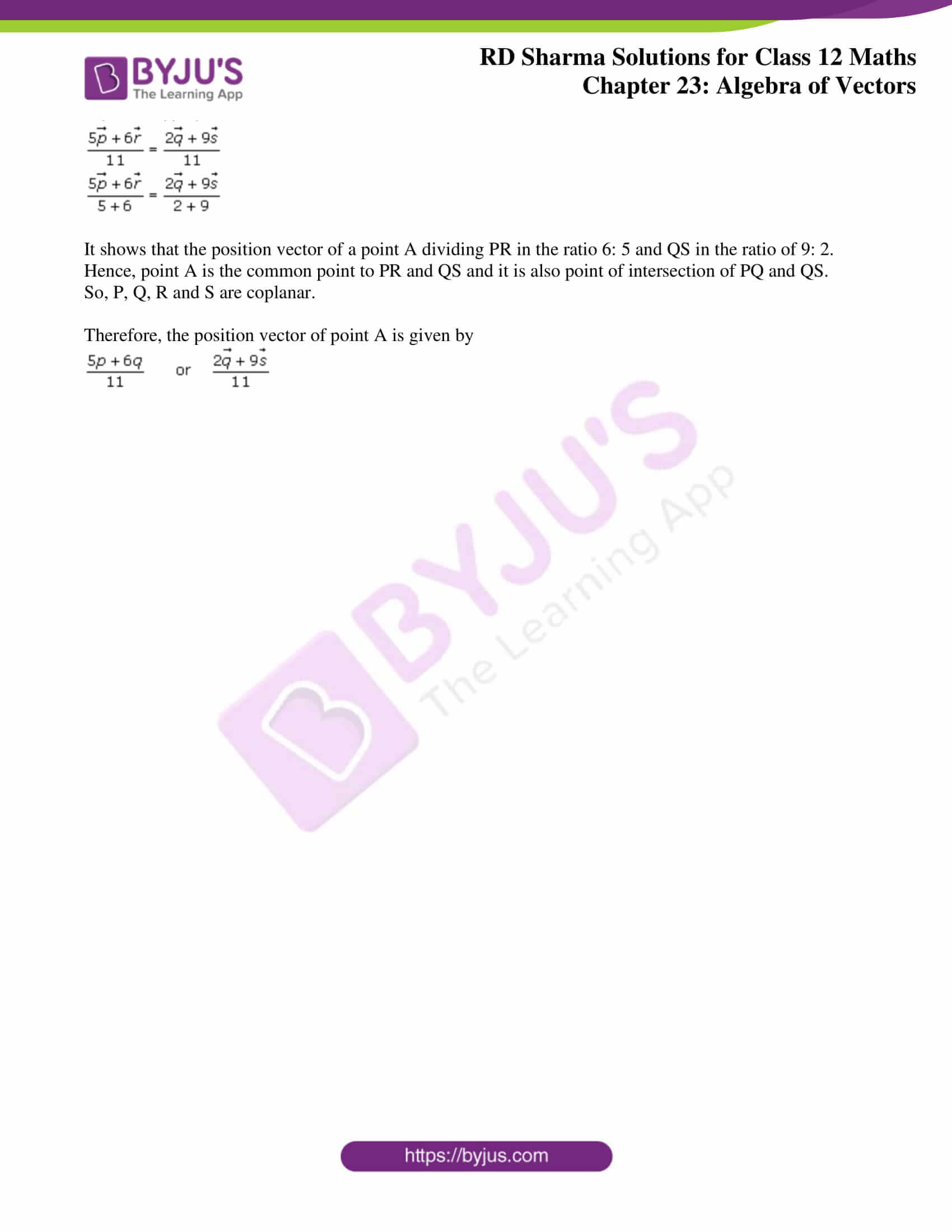### Access Answers for Rd Sharma Solution Class 12 Maths Chapter 23 Exercise 3

Exercise 23.3

1. Solution:

When,

Point R divides the line joining the two points P and Q in the ratio 1: 2 internally.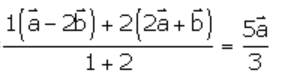So, position vector of point R =

And when,

Point R divides the line joining the two points P and Q in the ratio 1: 2 externally.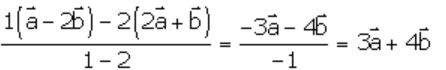So, position vector of point R =

2. Solution:

It’s given that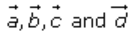be the position vectors of the four distinct points A, B, C and D such that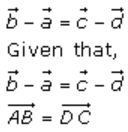So, AB is parallel and equal to DC (in magnitude).

Therefore, ABCD is a parallelogram.

3. Solution:

It’s given that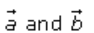are position vectors of A and B respectively.

Let C be a part in AB produced such that AC = 3AB

Thus, it’s clear that point C divides the line AB in ratio 3: 2 externally

So,

Position vector of point C is given by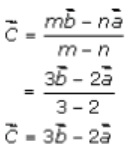Again, let D be a point in BA produced such that BD = 2BA

Let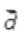be the position vector of D. It is clear that point D divides the line AB in 1: 2 externally. So, position vector of D is given by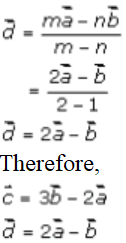4. Solution:

Given that,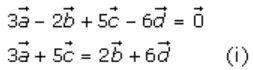It’s seen that the sum of the coefficients on both the sides of the equation (i) is 8, so divide equation (i) by 8 on both the sides.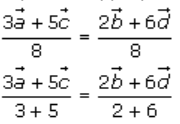It shows that the position vector of a point P dividing AC in the ratio 3: 5, is same as that of point dividing BD in the ratio of 2: 6.

Hence, point P is the common point to AC and BD and P is the point of intersection of AC and BD.

So, A, B, C and D are coplanar.

Therefore, the position vector of point P is given by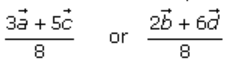5. Solution: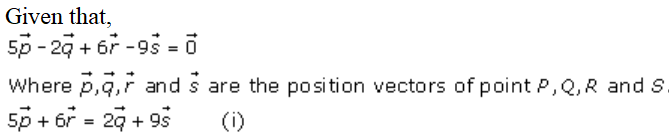It’s seen that the sum of the coefficients on both the sides of the equation (i) is 11, so divide equation (i) by 11 on both the sides.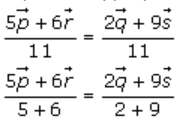It shows that the position vector of a point A dividing PR in the ratio 6: 5 and QS in the ratio of 9: 2.

Hence, point A is the common point to PR and QS and it is also point of intersection of PQ and QS.

So, P, Q, R and S are coplanar.

Therefore, the position vector of point A is given by